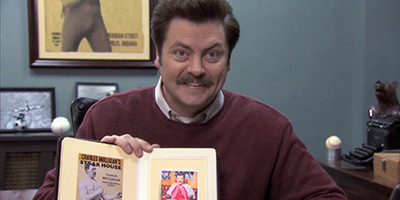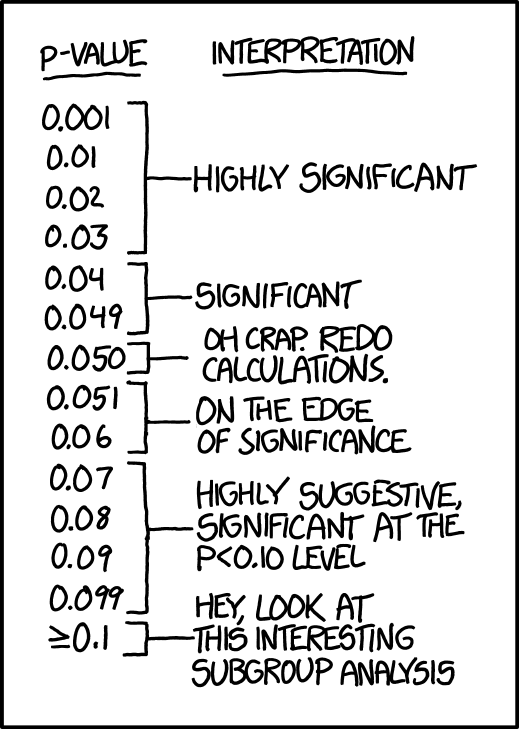# Exercise 1

Before it closed, Ron Swanson was a frequent patron of Charles Mulligan’s Steakhouse in Indianapolis, Indiana. Ron enjoyed the experience so much, during each visit he took a picture with his steak.Ron also weighed each steak he consumed. He has a record of eating six “22 ounce” Charles Mulligan’s porterhouse steaks. Ron found that these six steaks weighed

$22.4 \text{ oz}, \ 20.8 \text{ oz}, \ 21.6 \text{ oz}, \ 20.2 \text{ oz}, \ 21.4 \text{ oz}, \ 22.0 \text{ oz}$

Suppose that the weight of “22 ounce” Charles Mulligan’s porterhouse steaks follow a $$N(\mu, \sigma^2)$$ distribution and that Ron’s six steaks were a random sample.

Ron is suspicious of this “22 ounce” claim. Use this sample to test

$H_0: \mu = 22 \text{ versus } H_1: \mu < 22$

Report:

• The test statistic
• The crictical value when $$\alpha = 0.05$$.
• The decision when $$\alpha = 0.05$$.

# Exercise 2

Let’s hope that Exercise 1 fails to reject, and the steaks could really have a mean of 22 ounces. But how variable are they? If the variance is too high, Ron might end up with a really small steak on a particular visit.

Use the sample from Exercise 1 to test

$H_0: \sigma = 0.50 \text{ versus } H_1: \sigma > 0.50$

Report:

• The test statistic
• The crictical value when $$\alpha = 0.01$$.
• The decision when $$\alpha = 0.01$$.

# Exercise 3

Last year, ballots in Champaign-Urbana contained the following question to assess public opinion on an issue:

“Should the State of Illinois legalize and regulate the sale and use of marijuana in a similar fashion as the State of Colorado?”

Suppose that we would like to understand Champaign-Urbana’s 2017 opinion on marijuana legalization. To satisfy our curiosity, we obtain a random sample of 120 Champaign-Urbanians and find that 87 support marijuana legalization.

Calculate the p-value of the test

$H_0: p = 0.70 \text{ versus } H_1: p > 0.70$

where $$p$$ is the true proportion of Champaign-Urbanians that support marijuana legalization.

Do this two ways:

• Using the approximate large-sample $$z$$ procedure seen in class.
• Using an exact test.

For the exact test, calculate the probability of seeing as many supporters as observed, or more, assuming the null hypothesis is true. (Hint: Use a binomial distribution. Also, consider doing the calculation in R.)

# Exercise 4

Last year, ballots in Champaign-Urbana contained the following question to assess public opinion on an issue:

“Should the State of Illinois legalize and regulate the sale and use of marijuana in a similar fashion as the State of Colorado?”

Suppose we obtain a random sample of 80 Champaign voters, of which 55 support marijuana legalization. We also obtain a random sample of 100 Urbana voters, of which 75 support marijuana legalization. Let $$p_C$$ be the true proportion of Champaign voters who support marijuana legalization and let $$p_U$$ be the true proportion of Urbana voters who support marijuana legalization.

Perform a test of

$H_0: p_U = p_C \text{ versus } H_1: p_U \neq p_C$

Report:

• The test statistic
• The p-value
• The decision when $$\alpha = 0.05$$.

# Exercise 5

On January 18, 2015, Clete Blakeman measured the pressure in pounds per square inch (PSI) of 15 footballs during halftime of the AFC Championship game. Of these footballs, 11 were a sample from the New England Patriots. The remaining 4 were a sample from the Indianapolis Colts. The data follows:

pats  = c(11.50, 10.85, 11.15, 10.70, 11.10, 11.60, 11.85, 11.10, 10.95, 10.50, 10.90)
colts = c(12.70, 12.75, 12.50, 12.55)

Assume these are both random samples from a normal distribution with equal variances. Use this data to test:

$H_0: \mu_P = \mu_C \text{ versus } H_1: \mu_P \neq \mu_C$

where $$\mu_P$$ is the mean PSI of the Patriot’s footballs and $$\mu_C$$ is the mean PSI of the Colt’s footballs.

Report:

• The test statistic
• The p-value
• You’ll need to use R for this calculation. In particular, the pt() function.
• The result will be really, really small because the Patriot’s are dirty cheaters!! OK, I shouldn’t be so certain. Also this analysis is a little simplified. For actual details, see the Wells Report. (Note that the report contains text messages that use some not so pleasant language.)
• The decision when $$\alpha = 0.01$$.